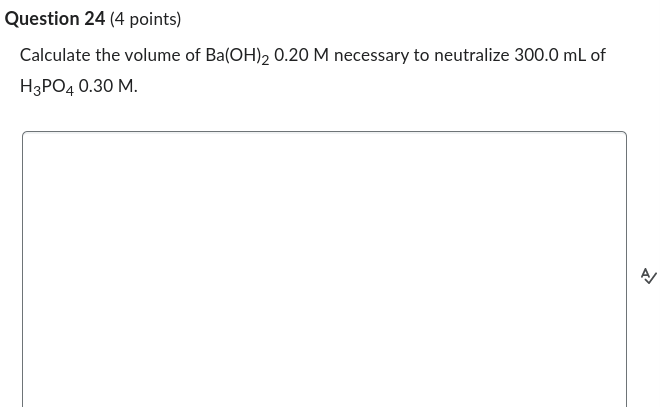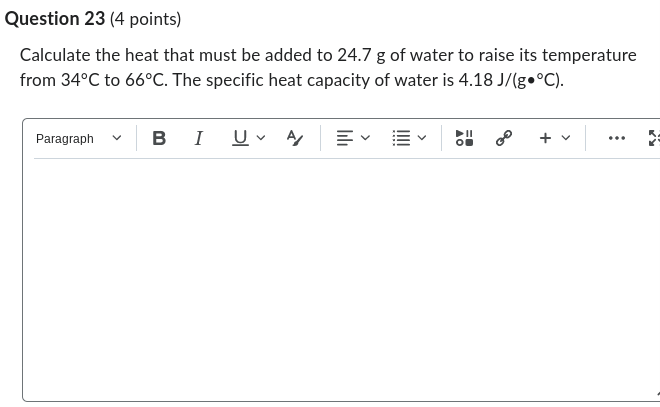# Question Solved1 Answer Question 24 (4 points) Calculate the volume of Ba(OH)2 0.20 M necessary to neutralize 300.0 mL of H3PO4 0.30 M. 2 Question 23 (4 points) Calculate the heat that must be added to 24.7 g of water to raise its temperature from 34°C to 66°C. The specific heat capacity of water is 4.18 J/(g•°C). Paragraph B I UVA/ 영 + v Question 24 (4 points) Calculate the volume of Ba(OH)2 0.20 M necessary to neutralize 300.0 mL of H3PO4 0.30 M. 2 Question 23 (4 points) Calculate the heat that must be added to 24.7 g of water to raise its temperature from 34°C to 66°C. The specific heat capacity of water is 4.18 J/(g•°C). Paragraph B I UVA/ 영 + vTranscribed Image Text: Question 24 (4 points) Calculate the volume of Ba(OH)2 0.20 M necessary to neutralize 300.0 mL of H3PO4 0.30 M. 2 Question 23 (4 points) Calculate the heat that must be added to 24.7 g of water to raise its temperature from 34°C to 66°C. The specific heat capacity of water is 4.18 J/(g•°C). Paragraph B I UVA/ 영 + v
More
Transcribed Image Text: Question 24 (4 points) Calculate the volume of Ba(OH)2 0.20 M necessary to neutralize 300.0 mL of H3PO4 0.30 M. 2 Question 23 (4 points) Calculate the heat that must be added to 24.7 g of water to raise its temperature from 34°C to 66°C. The specific heat capacity of water is 4.18 J/(g•°C). Paragraph B I UVA/ 영 + v